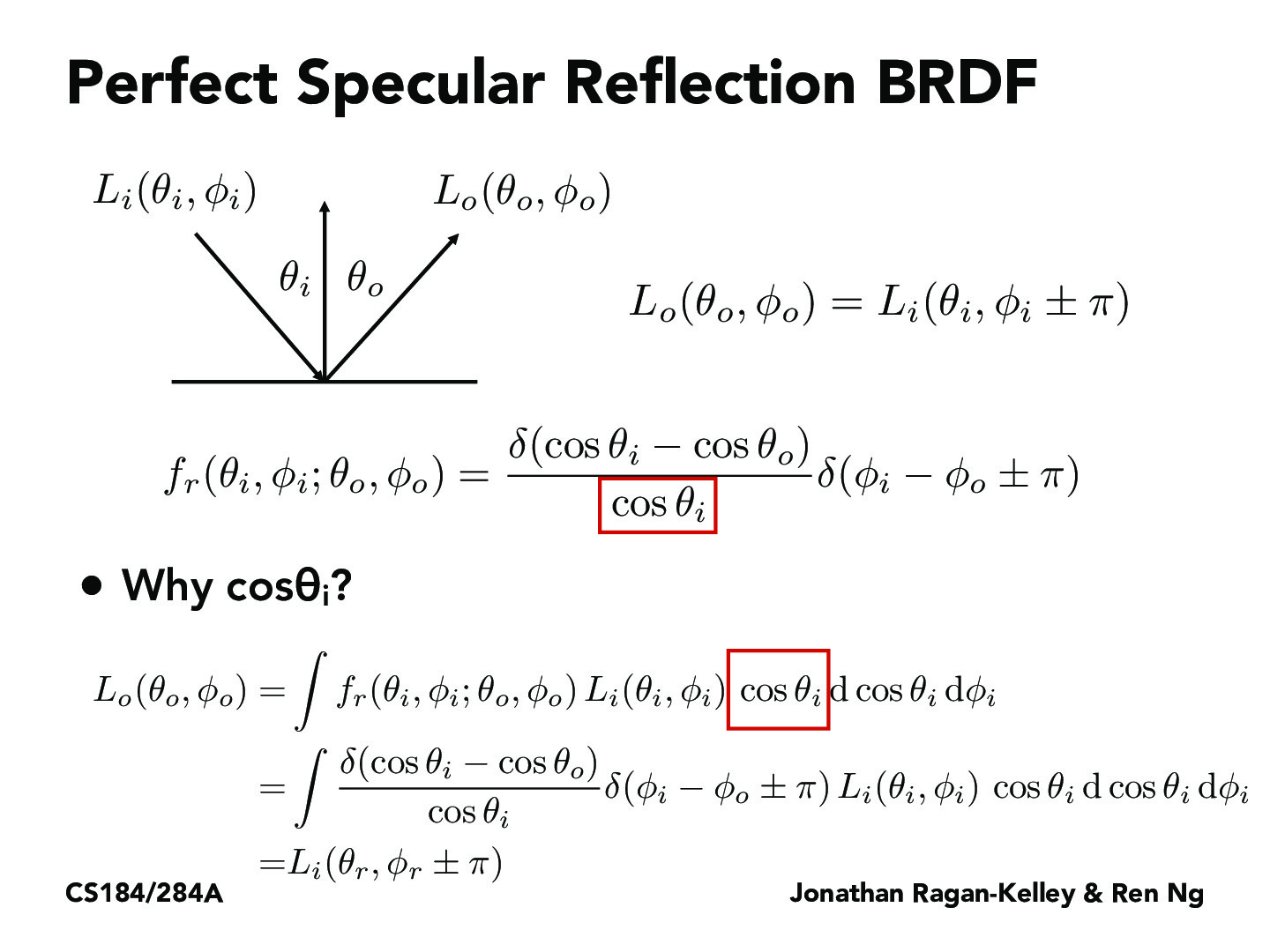You are viewing the course site for a past offering of this course. The current offering may be found here.Lecture 14: Material Modeling (17)JerryJiehanWang

Why d(cos(theda)_i - cos(theta)_o) will gives us everywhere else is 0 except cos(theta)_o?sirejdua

Here, the delta function is referring to the indicator function. delta(x) = 1 when x = 0, and delta(x) = 0 else. So, delta(cos(theta_i) - cos(theta_o)) is 0 whenever cos_theta(i) - cos(theta_o) != 0.eric99ying

What is the theta_r in the final term at the bottom? Is it the sum of theta_i and theta_o?dtseng

I think you're referring to \phi_r, and I think they meant \phi_i instead of \phi_r. The first equation is the statement this entire slide is about, and the last equation is just an expansion of that equation.rahulmalayappan

In the bottom expression, theta_r is just the reflected theta value; it's just theta_o, I'm pretty sure.

You must be enrolled in the course to comment